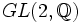# General linear group:GL(2,Q)

This group, denoted$GL(2,\mathbb{Q})$ or$GL_2(\mathbb{Q})$, is defined as the general linear group of degree two over the field of rational numbers.